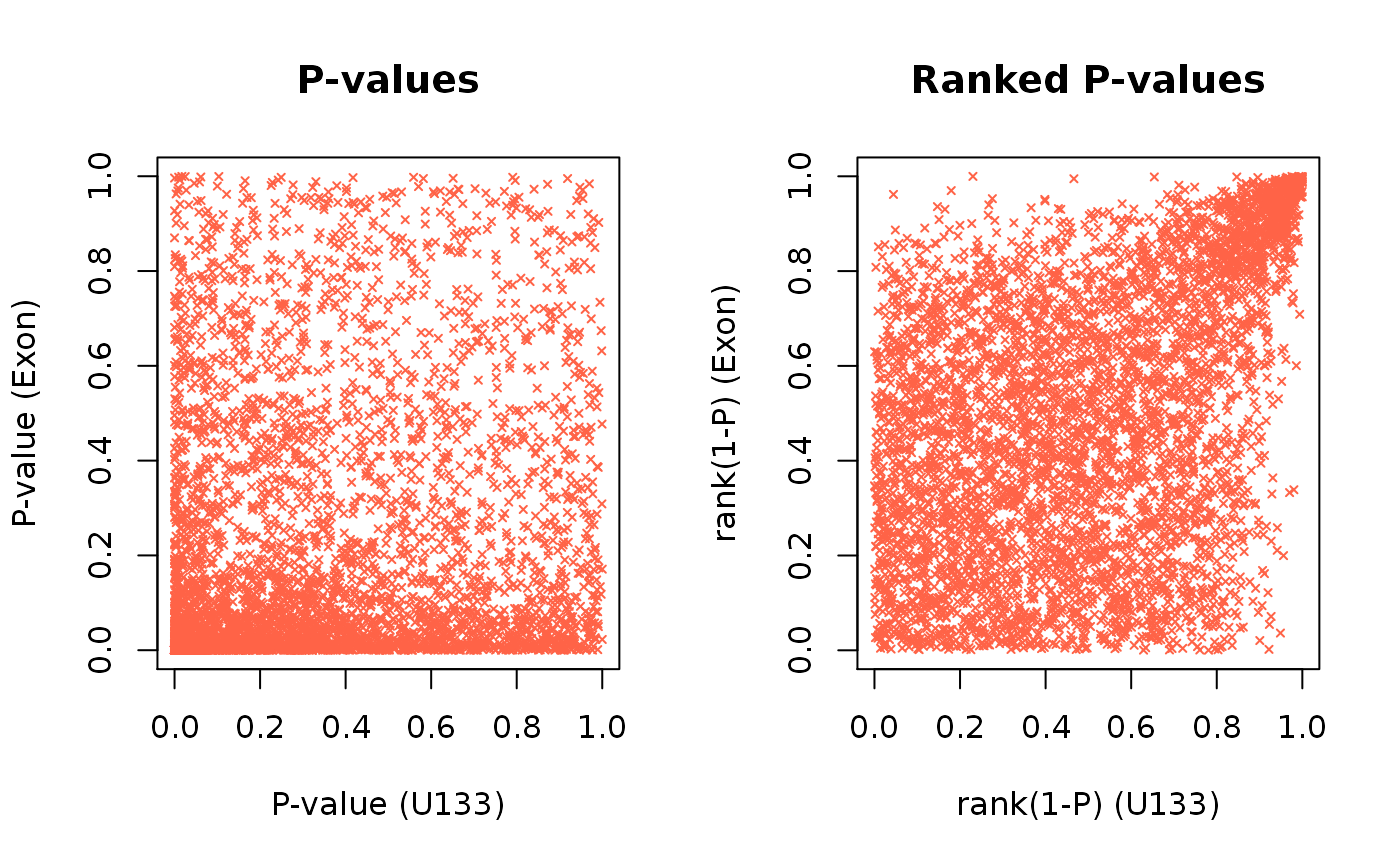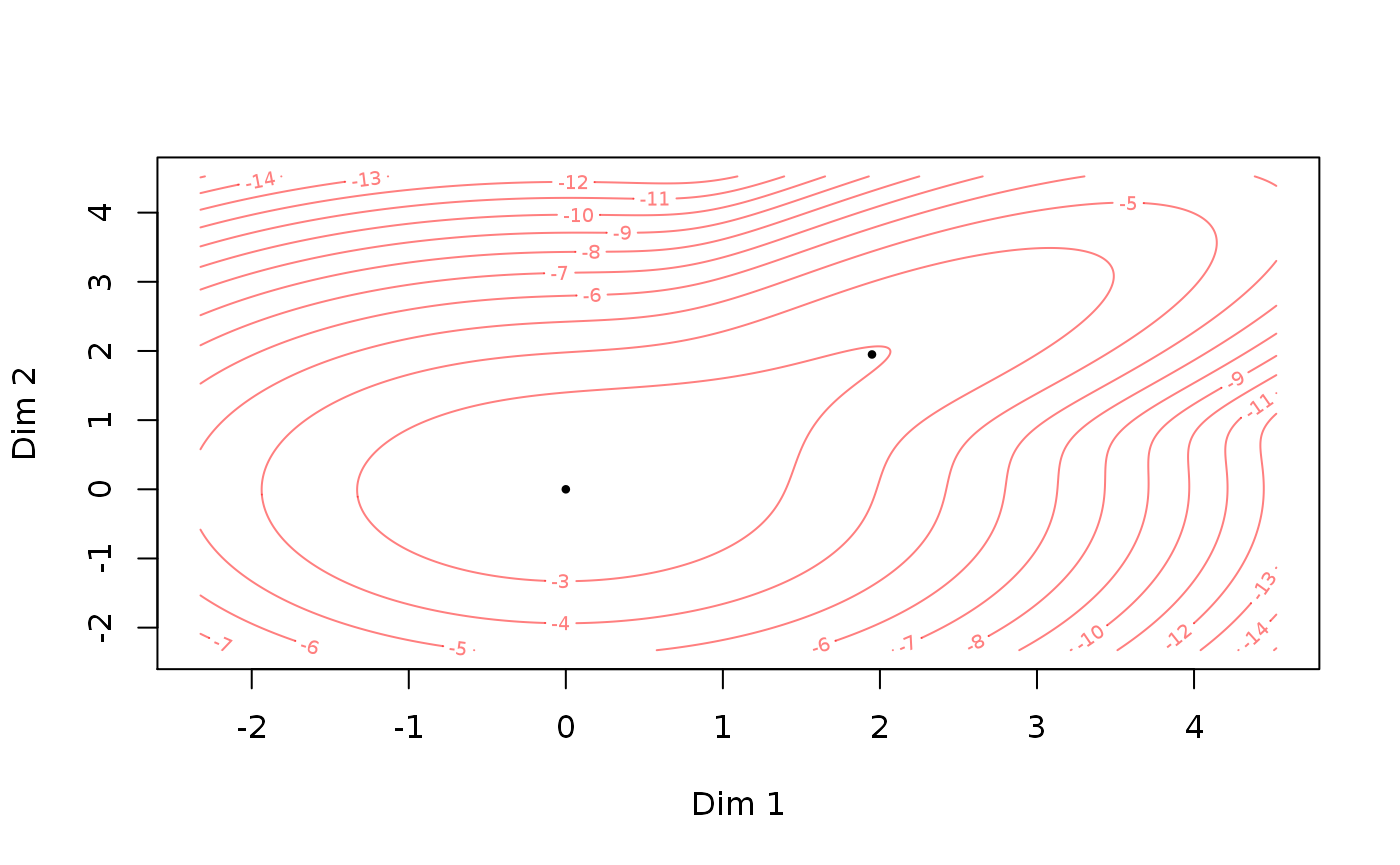This is a quick tutorial for using the special GMCM for meta analysis.

## Initialization

#install.packages("GMCM")  # Uncomment to install the GMCM package
library("GMCM")

If GMCM is not installed, please uncomment the above line and rerun the script.

The data is loaded and the first rows are printed. To illustrate we load the u133VsExon dataset within the package. The dataset contains 19,577 p-values for the null hypothesis of no differential gene expression between two cell types for each of two different experiments called u133 and exon.

x <- get(data("u133VsExon"))
head(x, n = 4)
##                         u133         exon
## ENSG00000265096 0.1756103672 1.072572e-01
## ENSG00000152495 0.0017797571 6.741108e-10
## ENSG00000198040 0.0053705738 1.505019e-03
## ENSG00000229092 0.0006693361 6.755118e-05

See help("u133VsExon") for more information.

Next, we subset the data to simply reduce computation time. After that, we will rank it, and visualize it.

x <- x[1:5000, ]

The values above are p-values where small values indicate strong evidence—contrary to what is expected by the special model. In the special model, large values should be critical to the null hypothesis.

Therefore we ranks and scale 1 - p:

u <- Uhat(1 - x)

The original and ranked data is plotted:

par(mfrow = c(1,2))  # Visualizing P-values and the ranked P-values
plot(x, cex = 0.5, pch = 4, col = "tomato", main = "P-values",
xlab = "P-value (U133)", ylab = "P-value (Exon)")
plot(u, cex = 0.5, pch = 4, col = "tomato", main = "Ranked P-values",
xlab = "rank(1-P) (U133)", ylab = "rank(1-P) (Exon)")Here each point represent a gene. The genes in the lower left of the first panel and correspondingly in the upper right of the second panel are the seemingly reproducible genes. They have a low p-value and thus a high rank in both experiments. The genes in the upper left and lower right are the ones that are apparently spurious results; they have a low p-value in only one experiment.

## Initial parameters

The initial parameters are set to

init_par <- c(pie1 = 0.6, mu = 1, sigma = 1, rho = 0.2)

## Model fitting

With the data loaded and prepared, the model is can be fitted with the defined initial parameters.

par <- fit.meta.GMCM(u = u,
init.par = init_par,
method = "NM",
max.ite = 1000,
verbose = FALSE)
print(par)
##      pie1        mu     sigma       rho
## 0.7489036 1.9494403 1.1066673 0.7360319

We here use the Nelder-Mead fitting method with with a maximum number of iterations of 1000.

## Meta analysis with unsupervised clustering

The estimated parameters are used to calculate the local and adjusted irreproducibility discovery rates:

meta_IDR_thres <- 0.05
out <- get.IDR(x, par = par,
threshold = meta_IDR_thres) # Compute IDR
str(out)
## List of 5
##  $idr : num [1:5000] 0.872 0.99 0.976 0.981 0.203 ... ##$ IDR      : num [1:5000] 0.4894 0.769 0.7032 0.7252 0.0645 ...
##  $l : int 431 ##$ threshold: num 0.05
##  $Khat : num [1:5000] 1 1 1 1 1 1 1 1 1 1 ... out <- get.IDR(u, par = par, threshold = meta_IDR_thres) below <- out[["IDR"]] < meta_IDR_thres out$l <- sum(below)
out$Khat <- ifelse(below, 2, 1) By default, get.IDR computes thresholds on the adjusted IDR. The local irreproducibility discovery rate correspond to the posterior probability of the point originating from the irreproducible component. ## Results The classes are counted by table(out$Khat)
##
##    1    2
## 4136  864

Where we see how many of the 5000 genes are deemed reproducible and irreproducible.

The results are also displayed by plotting

plot(x, col = out$Khat, asp = 1) # Plot of raw valuesplot(u, col = out$Khat, asp = 1) # Plot of copula valuesz <- GMCM:::qgmm.marginal(u, theta = meta2full(par, d = ncol(u))) # Estimate latent process
plot(z, col = out\$Khat, asp = 1) # Plot of estimated latent processThe model indeed capture genes in the upper right and classify them as reproducible.

The fitted par object can be converted to a theta object which can be plotted directly:

plot(meta2full(par, d = ncol(u)))### Session information

This report was generated using rmarkdown2 and knitr3 under the session given below. The report utilizes parameterized reports and knitr::spin.

sessionInfo()
## R version 4.0.2 (2020-06-22)
## Platform: x86_64-pc-linux-gnu (64-bit)
## Running under: Ubuntu 16.04.6 LTS
##
## Matrix products: default
## BLAS:   /usr/lib/openblas-base/libblas.so.3
## LAPACK: /usr/lib/libopenblasp-r0.2.18.so
##
## locale:
##   LC_CTYPE=en_US.UTF-8       LC_NUMERIC=C
##   LC_TIME=en_US.UTF-8        LC_COLLATE=en_US.UTF-8
##   LC_MONETARY=en_US.UTF-8    LC_MESSAGES=en_US.UTF-8
##   LC_PAPER=en_US.UTF-8       LC_NAME=C
##  LC_MEASUREMENT=en_US.UTF-8 LC_IDENTIFICATION=C
##
## attached base packages:
##  stats     graphics  grDevices utils     datasets  methods   base
##
## other attached packages:
##  GMCM_1.4.1 knitr_1.30
##
## loaded via a namespace (and not attached):
##   Rcpp_1.0.5         digest_0.6.27      crayon_1.3.4       rprojroot_1.3-2
##   assertthat_0.2.1   R6_2.5.0           backports_1.2.0    magrittr_1.5
##   ellipse_0.4.2      evaluate_0.14      stringi_1.5.3      rlang_0.4.8
##  fs_1.5.0           ragg_0.3.1         rmarkdown_2.5      pkgdown_1.6.1.9000
##  desc_1.2.0         tools_4.0.2        stringr_1.4.0      yaml_2.2.1
##  xfun_0.19          compiler_4.0.2     systemfonts_0.3.1  cpp11_0.2.1
##  memoise_1.1.0      htmltools_0.5.0